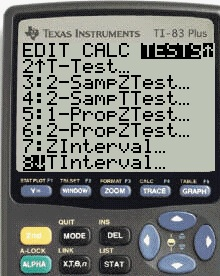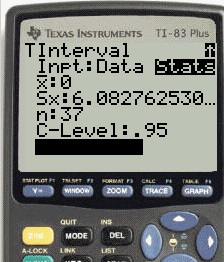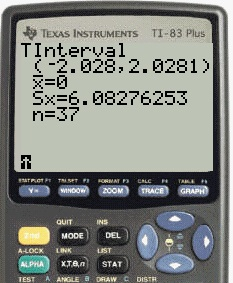# How to Find t Critical Value on TI 83

Share on

Probability and Statistics > TI-83 for Statistics > how to find t critical value on ti 83

Watch the video for an example:

IMPORTANT: The TI-83 doesn’t have a function to calculate the t critical value directly. In other words, you won’t find it listed on a menu as something like “invT”. However, you can calculate it; it just takes a couple of extra steps.

• if you want a t critical value for a two tailed test, follow the steps below. The confidence interval limits are the same as the t critical values.
• if you want the t critical value for a one tailed test, you can’t use the TI83 unless you program it (see this video for instructions ).

## How to Find T Critical Value on TI 83: Steps

Sample Question: Calculate the two-talied t-critical value for 36 degrees of freedom (df = 36) for an alpha level of 0.05 (α = 0.05).

Step 1: Press the “STAT” key, then press the left arrow key to arrive at the Tests menu.Step 2: Press “8” for TInterval.

Step 3: Arrow right to choose STATS. Press ENTER.

Step 3: Change the values in the list. For this sample question:• Set the mean (Xbar) to 0
• Set Sx to the square root of your sample size. The sample size is df+1, so for this example press √37.
• Set n as your sample size. For this example, it’s 37.
• Set your confidence level, which is 1 – α For this example, set it to .95.

Step 4: Highlight CALCULATE and then press Enter. The t-critical value is The same as the given confidence level limits: 2.028.CITE THIS AS:
Stephanie Glen. "How to Find t Critical Value on TI 83" From StatisticsHowTo.com: Elementary Statistics for the rest of us! https://www.statisticshowto.com/find-t-critical-value-ti-83/
---------------------------------------------------------------------------Need help with a homework or test question? With Chegg Study, you can get step-by-step solutions to your questions from an expert in the field. Your first 30 minutes with a Chegg tutor is free!